# Solution assignment 08 Root equations

### Assignment 8

Solve: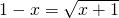### Solution

We can first try to write the conditions which must be met for which this equation is defined. We can also begin solving the equation and check afterwards whether solutions satisfy the equation. We now choose the second approach.
We square both sides of the equation: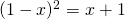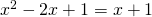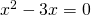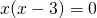This equation has two solutions: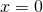or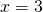Now we check whether these solutions satisfy the original equation. The first solution satisfies the equation, the second does not.
Thus the solution of the original equation is: